## Factor the polynomial below. x2 + 6x + 9

Question

Factor the polynomial below.

x2 + 6x + 9

in progress 0
3 weeks 2021-08-23T23:40:34+00:00 2 Answers 0 views 0

(x-3) is the factor of polynomial x2 + 6x + 9

and it’s zero is -3

Step-by-step explanation:

by solving it we get ;

=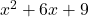=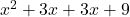=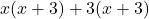=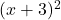now, equating it with 0, we get

=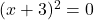=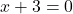=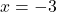so, the factor is ( x + 3 )